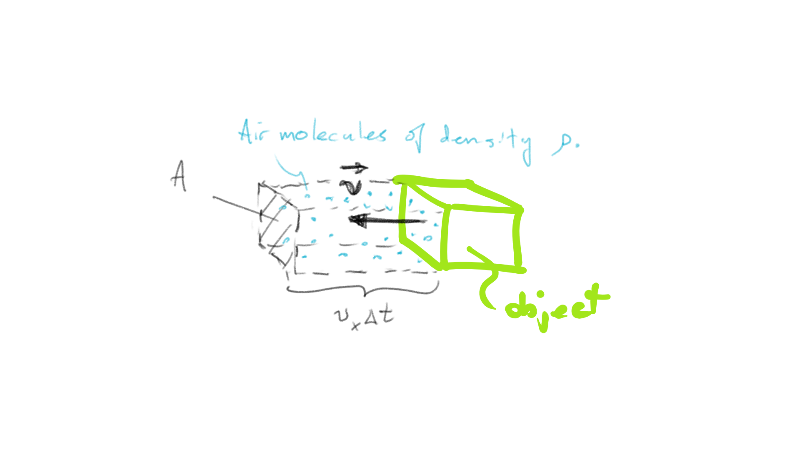# Air Drag DerivationAn object with frontal area A, moving at speed v in the x-direction through air of density .

## Collisions with Molecules

To derive an expression for the drag force an object experiences as it moves through air, we will look at the individual collisions between air molecules and the front of an object. When they collide, the average force can be found using Newton's second law: Clearly we have lots of short-lived collisions between individual molecules with the front of the object moving through the air. In that sense, the air drag is an average force delivered over time by those collisions. So we should rewrite Newton's second law as average force: The mass in this equation is the mass of all the air molecules collided with over time Written in terms of density and volume occupied by the molecules encountered in that time duration we can write It should be noted that at speeds rather slow compared to the speed of sound in air (~340 m/s) that only half of the molecules in any volume will strike the front surface of the object moving through the air. That is because thermal motion will be, on average, carrying half of the molecules away from the moving object at a speed much greater than it is chasing the molecules since v<<340m/s. The symbol << means "much less than" to a physicist if you haven't seen it before. Given this fact, we write The next manipulation is to recognize that the volume encountered in a time is equal to the frontal area of the object times the distance that it travels in time That distance is of course if we assume it's moving in the x-direction. Mathematically then, we get Plugging this into the average force equation and assuming we are moving in the x-direction, and therefore experience a force along that same direction, gives us:

We will cancel the time interval and assume the change in velocity is or all the speed of incoming air (from the object's perspective) is lost upon collision. That gives us:

The last consideration is to account for situations where the air is slowed but not stopped. To account for this we add a drag coefficient. Lower means less flow disruption. In principle it could be as large as 2 since that would send the air recoiling as fast as it arrived upon collision like an elastic ball bouncing. In general this doesn't happen often since energy is lost upon colliding which leads to a slight temperature increase of the moving object. On the other hand, if more air is disrupted than that which is accounted for by the frontal area, the drag coefficient can be inflated a bit. If you look at measured values it tends to be limited by The final expression for drag is:

As we saw in an earlier section, the vectorized version is written: